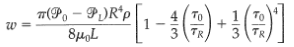### Create an Account

Home / Questions / Derivation of the Buckingham Reiner equation2rework Example 8 3 1 for the Bingham model fi...

# Derivation of the Buckingham Reiner equation2rework Example 8 3 1 for the Bingham model first find the velocity distribution Then show that the mass rate of flow is given by in which

Derivation of the Buckingham-Reiner equation2rework Example 8.3-1 for the Bingham model, first find the velocity distribution. Then show that the mass rate of flow is given by in which tR = (P0 – PL)2L is the shear stress at the tube wall. This expression is valid only tR <>Jul 25 2020 View more View LessSubscribe To Get Solution